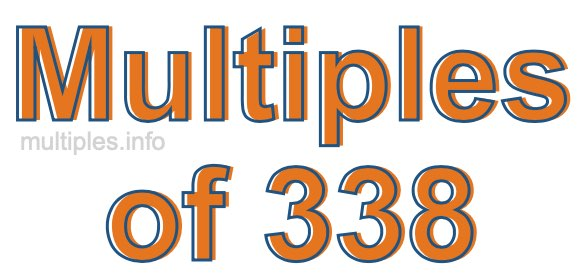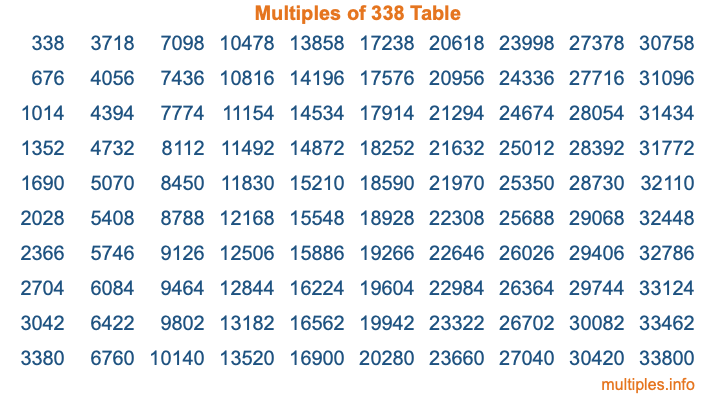Multiples of 338Welcome to the Multiples of 338 page. Here we will first teach you everything you will ever need to know about the multiples of 338, and then give you a study guide summary of everything we taught you to make sure you remember it all. Use this page to look up facts and learn information about the multiples of 338. This page will make you a multiples of three hundred thirty-eight expert!

Definition of Multiples of 338
Multiples of 338 are all the numbers that when divided by 338 equal an integer. Each of the multiples of 338 are called a multiple. A multiple of 338 is created by multiplying 338 by an integer.

Therefore, to create a list of multiples of 338, you start with 1 multiplied by 338, then 2 multiplied by 338, then 3 multiplied by 338, and so on for as long as you want. Thus, the list of the first five multiples of 338 is 338, 676, 1014, 1352, and 1690. To see a larger list of multiples of 338, see the printable image of Multiples of 338 further down on this page. We also have a category where you can choose any nth multiple of 338.

Multiples of 338 Checker
The Multiples of 338 Checker below checks to see if any number of your choice is a multiple of 338. In other words, it checks to see if there is any number (integer) that when multiplied by 338 will equal your number. To do that, we divide your number by 338. If the the quotient is an integer, then your number is a multiple of 338.

Is  a multiple of 338?

Least Common Multiple of 338 and ...
A Least Common Multiple (LCM) is the lowest multiple that two or more numbers have in common. This is also called the smallest common multiple or lowest common multiple and is useful to know when you are adding our subtracting fractions. Enter one or more numbers below (338 is already entered) to find the LCM.

Check out our LCM Calculator if you need more details about the Least Common Multiple or if you need the LCM for different numbers for adding and subtraction fractions.

nth Multiple of 338
As we stated above, 338 is the first multiple of 338, 676 is the second multiple of 338, 1014 is the third multiple of 338, and so on. Enter a number below to find the nth multiple of 338.

th multiple of 338

Multiples of 338 vs Factors of 338
338 is a multiple of 338 and a factor of 338, but that is where the similarities end. All postive multiples of 338 are 338 or greater than 338. All positive factors of 338 are 338 or less than 338.

Below is the beginning list of multiples of 338 and the factors of 338 so you can compare:

Multiples of 338: 338, 676, 1014, 1352, 1690, etc.

Factors of 338: 1, 2, 13, 26, 169, 338

As you can see, the multiples of 338 are all the numbers that you can divide by 338 to get a whole number. The factors of 338, on the other hand, are all the whole numbers that you can multiply by another whole number to get 338.

It's also interesting to note that if a number (x) is a factor of 338, then 338 will also be a multiple of that number (x).

Multiples of 338 vs Divisors of 338
The divisors of 338 are all the integers that 338 can be divided by evenly. Below is a list of the divisors of 338.

Divisors of 338: 1, 2, 13, 26, 169, 338

The interesting thing to note here is that if you take any multiple of 338 and divide it by a divisor of 338, you will see that the quotient is an integer.

Multiples of 338 Table
Below is an image of the first 100 multiples of 338 in a table. The table is in chronological order, column by column. The first column has the first ten multiples of 338, the second column has the next ten multiples of 338, and so on.The Multiples of 338 Table is also referred to as the 338 Times Table or Times Table of 338. You are welcome to print out our table for your studies.

Negative Multiples of 338
Although not often discussed or needed in math, it is worth mentioning that you can make a list of negative multiples of 338 by multiplying 338 by -1, then by -2, then by -3, and so on, to get the following list of negative multiples of 338:

-338, -676, -1014, -1352, -1690, etc.

Multiples of 338 Summary
Below is a summary of important Multiples of 338 facts that we have discussed on this page. To retain the knowledge on this page, we recommend that you read through the summary and explain to yourself or a study partner why they hold true.

There are an infinite number of multiples of 338.

A multiple of 338 divided by 338 will equal a whole number.

338 divided by a factor of 338 equals a divisor of 338.

The nth multiple of 338 is n times 338.

The largest factor of 338 is equal to the first positive multiple of 338.

338 is a multiple of every factor of 338.

338 is a multiple of 338.

A multiple of 338 divided by a divisor of 338 equals an integer.

338 divided by a divisor of 338 equals a factor of 338.

Any integer times 338 will equal a multiple of 338.

Multiples of a Number
Here you can get the multiples of another number, all with the same attention to detail as we did for multiples of 338 on this page.

Multiples of
Multiples of 339
Did you find our page about multiples of three hundred thirty-eight educational? Do you want more knowledge? Check out the multiples of the next number on our list!